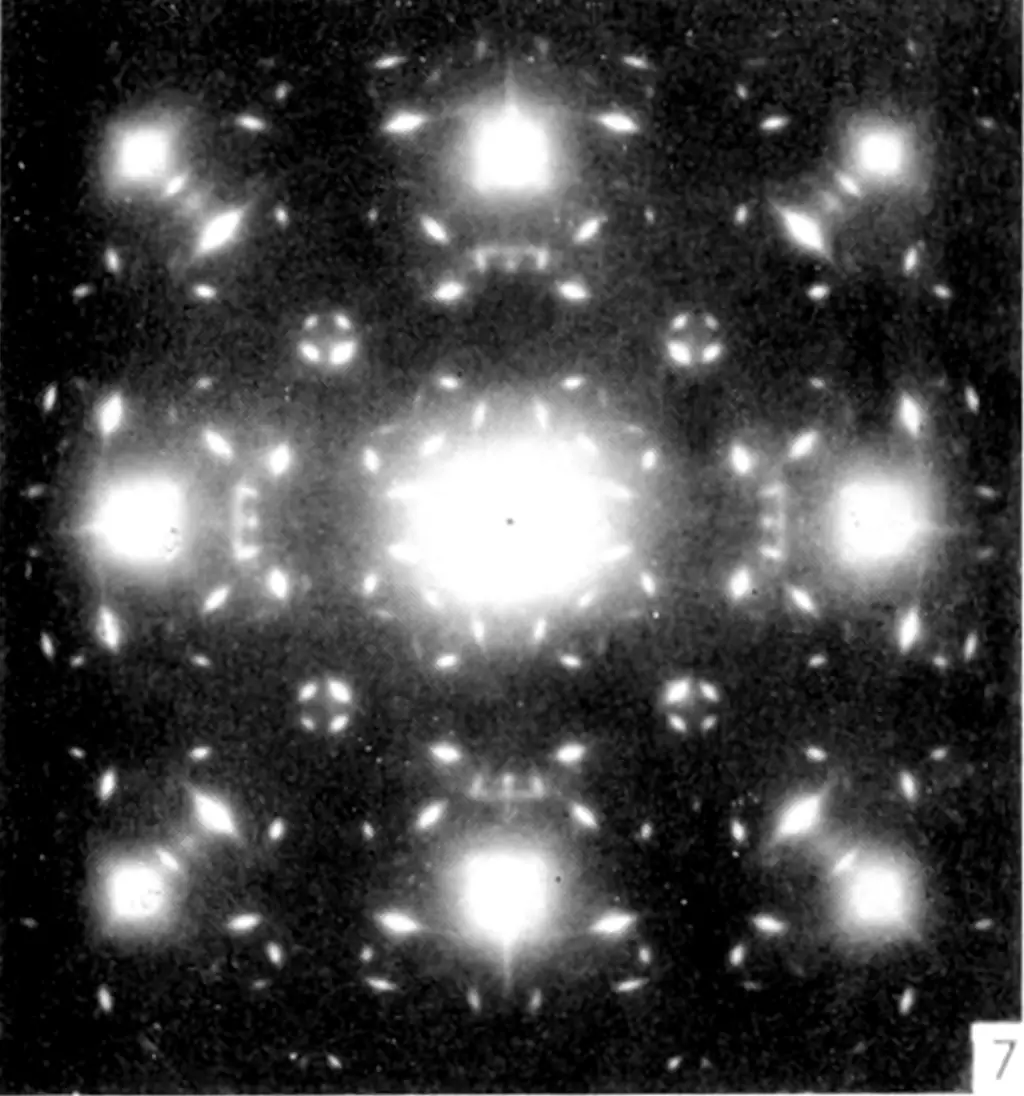# Last-layer Bayes neural nets

## Bayesian and other probabilistic inference in overparameterized ML## Last layer by (generalised) Bayesian linear regression

Classic in the modern setting: Snoek et al. (2015).

This looks interesting: Weber et al. (2018)

We propose a new method for training neural networks online in a bandit setting. Similar to prior work, we model the uncertainty only in the last layer of the network, treating the rest of the network as a feature extractor. This allows us to successfully balance between exploration and exploitation due to the efficient, closed-form uncertainty estimates available for linear models. To train the rest of the network, we take advantage of the posterior we have over the last layer, optimizing over all values in the last layer distribution weighted by probability. We derive a closed form, differential approximation to this objective and show empirically that this method leads to both better online and offline performance when compared to other methods

Haven’t seen it used much, which leads me to suspect there is some difficulty in practice.

## Last Layer Laplace

a.k.a. Neural Linear models.

AFAICT this case is the simplest. We are concerned with the density over the predictive, so we start with a neural network. Then we treat the neural network as a feature generator in all the layers up to the last one, and treat the last layer probabilistically, as an adaptive-basis regression or classification problem, to get a decent learnable predictive uncertainty. I think this was implicit in Mackay (1992), but it was named in Snoek et al. (2015), critiqued and extended in Lorsung (2021).

For a simple practical example, see the Probflow tutorial.

Under a last-layer Laplace approximation, we write the joint model as $$\vrv{y}= \vrv{r}^{\top}\Phi(\vrv{u})$$ so the joint distribution is \begin{align*} \left.\left[\begin{array}{c} \vrv{y} \\ \vrv{r} \end{array}\right]\right|\vrv{u} &\sim\dist{N}\left( \left[\begin{array}{c} \vv{m}_{\vrv{y}}\\ \vv{m}_{\vrv{r}} \end{array}\right], \left[\begin{array}{cc} \mm{K}_{\vrv{y}\vrv{y}} & \mm{K}_{\vrv{y}\vrv{r}}^{\top} \\ \mm{K}_{\vrv{y}\vrv{r}} & \mm{K}_{\vrv{r}\vrv{r}} \end{array}\right] \right) \end{align*} with \begin{align*} \vv{m}_{\vrv{y}} &=\vv{m}_{\vrv{r}}^{\top}\Phi(\vrv{u}) \\ \mm{K}_{\vrv{y}\vrv{r}} &=\Phi(\vrv{u}) \mm{K}_{\vrv{r}\vrv{r}}\\ \mm{K}_{\vrv{y}\vrv{y}} &= \Phi(\vrv{u})\mm{K}_{\vrv{r}\vrv{r}} \Phi^{\top} (\vrv{u})+ \sigma^2\mm{I}. \end{align*} Here $$\vrv{r}\sim \dist{N}\left(\vv{m}_{\vrv{r}}, \mm{K}_{\vrv{r}\vrv{r}}\right)$$ is the random weighting, and $$\Phi(\vrv{u})$$ is called the feature map.

## Last layer ensemble

TBD

### No comments yet. Why not leave one?

GitHub-flavored Markdown & a sane subset of HTML is supported.